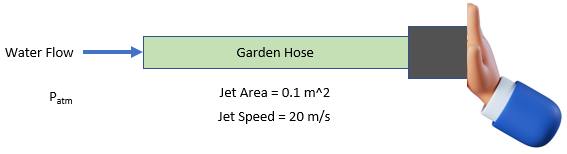## Garden Hose

Consider a typical garden hose produces a horizontal jet of water (density = 1,000 kg/m^3). Estimate the minimum force required to stop the water flow with your hand by pressing it perpendicularly against the nozzle's opening.Hint
$$Q=vA$$$where $$Q$$ is the flow rate, $$v$$ is the velocity, and $$A$$ is the cross sectional area. Hint 2 Since the water jet is deflected perpendicularly, the required force must deflect the total horizontal momentum of the water. $$F=\rho Qv$$$
where $$\rho$$ is the density, $$Q$$ is the flow rate, and $$v$$ is the fluid velocity.
Volumetric flow rate:
$$Q=vA$$$where $$Q$$ is the flow rate, $$v$$ is the velocity, and $$A$$ is the cross sectional area. $$Q=vA=(0.1m^2)(20m/s)=2\:m^3/s$$$
Since the water jet is deflected perpendicularly, the required force must deflect the total horizontal momentum of the water.
$$F=\rho Qv$$$where $$\rho$$ is the density, $$Q$$ is the flow rate, and $$v$$ is the fluid velocity. Thus, $$F=1,000\frac{kg}{m^3}\times 2\frac{m^3}{s} \times 20\frac{m}{s}=40,000\frac{kg\cdot m}{s^2}=40\:kN$$$
40 kN